# Swift Algorithm Club: Strassen’s Algorithm

In this tutorial, you’ll learn how to implement Strassen’s Matrix Multiplication in Swift. This was the first matrix multiplication algorithm to beat the naive O(n³) implementation, and is a fantastic example of the Divide and Conquer coding paradigm — a favorite topic in coding interviews.

#### Version

• Swift 4.2, iOS 12, Xcode 10

The Swift Algorithm Club, also known as SAC, is a community-driven open-source project that contributes to building popular algorithms and data structures in Swift.

Every month, the SAC team will feature a data structure or algorithm from the club in a tutorial on this site. If you want to learn more about algorithms and data structures, follow along with us!

Note: New to the Swift Algorithm Club? Check out the Getting Started post first.

This tutorial assumes you have read the following tutorials (or have equivalent knowledge):

## Getting Started

In this tutorial, you’ll learn how to implement Strassen’s Matrix Multiplication. It was developed by Volker Strassen, a German mathematician, in 1969 and was the first to beat the naive O(n³) implementation. In addition, Strassen’s algorithm is a fantastic example of the Divide and Conquer coding paradigm — a favorite topic in coding interviews.

Specifically, you will:

• Learn the applications of matrix multiplication and how it works.
• Implement a naive implementation.
• Implement Strassen’s algorithm and learn how it differs from the naive approach.

But, first, a little theory!

### Understanding Matrix Multiplication

Matrix multiplication is an operation that combines two matrices together. It is incredibly useful, having applications across physics, engineering and mathematics! Some real-world examples include:

2. Graph computations
3. Physical simulations

### How Does It Work?

To see how matrix multiplication works, consider the following example:To start, you’re multiplying two 2×2 matrices A and B.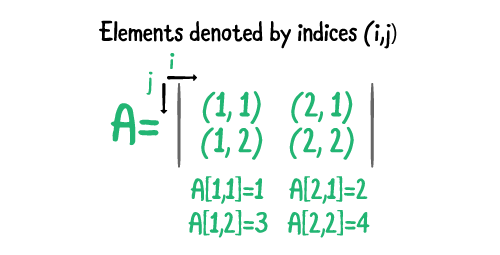Each element of matrix A is denoted by a combination of the indices (i, j). Both i and j start from 1 at the top-left corner of the matrix and increase by one until the final row and column. You denote the element at row i and column j by writing A[i, j]. Because matrix A has two rows and two columns, there are four index combinations:

1. A[1, 1] is equal to the element at the first row (i = 1) and the first column (j = 1).
2. A[2, 1] is the element at the second row (i = 2) and the first column (j = 1).
3. A[1, 2] is the element at the first row (i = 1) and the second column (j = 2).
4. A[2, 2] is the element at the second row (i = 2) and the second column (j = 2).

### Challenge

Write out each combination of indices i and j for matrix B. Include the element at each combination.

[spoiler title=”Solution”]

```B[1, 1] = 5
B[1, 2] = 6
B[2, 1] = 7
B[2, 2] = 8
```

[/spoiler]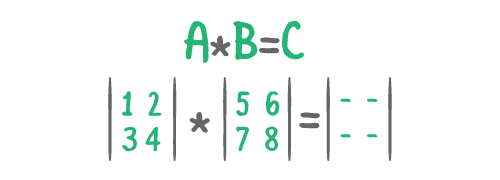The result of multiplying A and B is a new matrix: C. C’s elements are determined by taking the dot product of A’s i row with the B’s j column.

You’ll examine this operation in detail by filling in the four elements of C: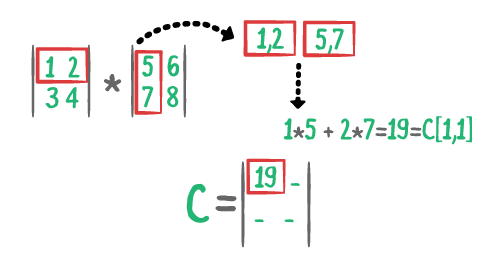1. [i=1, j=1]: The element at [1, 1] is the dot product of A’s first row and B’s first column. Because A’s first row is [1, 2] and B’s first column is [5, 7], you multiply 1 by 5 and 2 by 7. You then add the results together to get 19.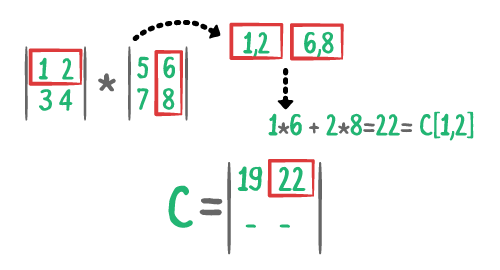2. [i=1, j=2]: Because i=1 and j=2, this component will represent the upper-right element in your new matrix, C. A’s first row is [1, 2] and B’s second column is [6, 8]. You multiply 1 by 6 and 2 by 8. You then add the results together to get 22.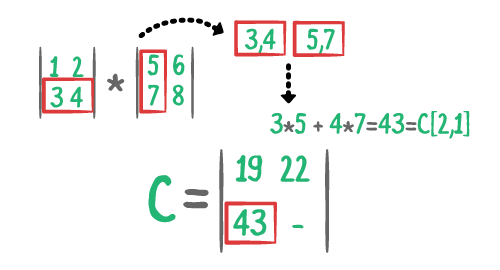3. [i=2, j=1]: A’s second row is [3, 4] and B’s first column is [5, 7]. Multiply 3 by 5 and 4 by 7; add those results together to get 43.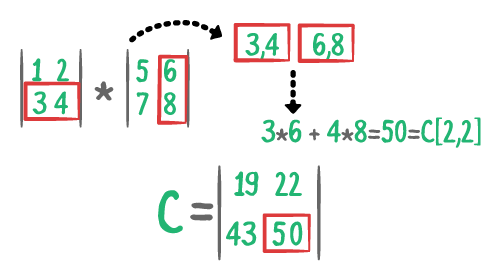4. [i=2, j=2]: A’s second row is [3, 4] and B’s second column is [6, 8]. By multiplying 3 by 6 and 4 by 8, then adding those results together, you get 50.

Phew, good job! That was a lot of work.

## Multiplying Matrices Correctly

For a matrix multiplication to be valid, the first matrix needs to have the same number of columns as the second matrix has number of rows. In math terminology, if matrix A’s dimensions are m × n, then matrix B’s dimensions need to be n × p. The result matrix C must be m × p. Look at an example in which this is not the case: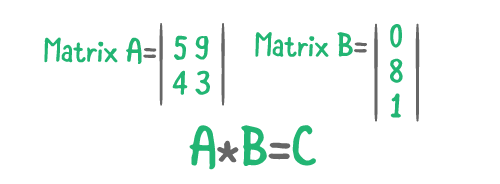You can use the same method as above: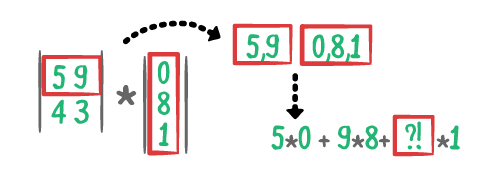1. [i=1, j=1]: A’s first row is [5, 9] and B’s first column is [0, 8, 1]. Multiply 5 by 0 and 9 by 8 and …?!?! There’s no third number from A’s first row to multiply by! You can check the result on WolfphramAlpha. This error is called incompatible dimensions. A’s first row and B’s first column are different lengths; you cannot take their dot product and therefore can’t complete the matrix multiplication.

Now for practice — try this next one on your own! :]

### Challenge

Multiply the following matrices:

```Matrix A:
1 8
9 0
21 -2

Matrix B:
5 2
2 4
```

[spoiler title=”Solution”]
[i=1, j=1]:

```A's first row = [1, 8]
B's first column = [5, 2]
[1, 8] dot [5, 2] = [1*5 + 8*2] =  = C[1, 1]```

[i=1, j=2]:

```A's first row = [1, 8]
B's second column = [2, 4]
[1, 8] dot [2, 4] = [1*2 + 8*4] =  = C[1, 2]```

[i=2, j=1]:

```A's second row = [9, 0]
B's first column = [5, 2]
[9, 0] dot [5, 2] = [9*5 + 0*2] =  = C[2, 1]```

[i=2, j=2]:

```A's second row = [9, 0]
B's second column = [2, 4]
[9, 0] dot [2, 4] = [9*2 + 0*4] =  = C[2, 2]```

[i=3, j=1]:

```A's third row = [21 -2]
B's first column = [5, 2]
[21, -2] dot [5, 2] = [21*5 + -2*2] =  = C[3,1]```

[i=3, j=2]:

```A's third row = [21, -2]
B's second column = [2, 4]
[21, -2] dot [2, 4] = [21*2 + -2*4] =  = C[3, 2]```
```Matrix C:
21 34
45 18
101 34
```

[/spoiler]

Aren’t you tired of doing matrix multiplication by hand? Let’s now look at how you can write this up in code!

## Naive Matrix Multiplication

Instead of dealing with implementing the specifics of a matrix in Swift, the starter project includes helper methods and a `Matrix` class to help you focus on learning matrix multiplication and Strassen’s algorithm.

Subscript Methods

• `subscript(row:column:)`: Returns the element at a specified row and column.
• `subscript(row:)`: Returns the row at a specified index.
• `subscript(column:)`: Returns the column at a specified index.

Term-by-Term Matrix Math

• `* (lhs:rhs:)`: Multiplies two matrices term-by-term.
• `+ (lhs:rhs:)`: Adds two matrices term-by-term.
• `- (lhs:rhs:)`: Subtracts two matrices term-by-term.

Array

• `dot(_:)`: Computes the dot product with a specified array and returns the result.

Functions

• `printMatrix(:name:)`: Prettily prints a specified matrix to the console.

For more details, look at `Matrix.swift` and `Array+Extension.swift`, both located under Sources. The file contains the implementation and documentation of all the methods and functions above!

### Implementing `matrixMultiply`

To begin implementing `matrixMultiply`, add a new extension to your playground with the following method:

```extension Matrix {
// 1
public func matrixMultiply(by other: Matrix) -> Matrix {
// 2
precondition(columnCount == other.rowCount,
"""
Two matrices can only be matrix multiplied if the first \
column's count is equal to the second's row count.
""")
}
}
```

1. You created an extension of `Matrix` with a public method named `matrixMultiply(by:)`.
2. Check to see if the current matrix’s column count matches the other matrix’s row count. Recall that this is a requirement, as highlighted when you learned about matrix multiplication.

Next, add the following to `matrixMultiply(by:)` below `precondition`:

```// 1
var result = Matrix(rows: rowCount, columns: other.columnCount)
// 2
for index in result.indices {
// 3
let ithRow = self[row: index.row]
let jthColumn = other[column: index.column]
// 4
result[index] = ithRow.dot(jthColumn)
}

return result
```

Going over what you just added, you:

1. Initialized the result matrix to have dimensions be the first matrix’s row count by the second matrix’s column count.
2. Looped through the indices of the matrix. `Matrix` is a `MutableCollection`, so this is exactly the same as looping through the indices of a regular `Array`.
3. Initialized constants for both the ith row from the first matrix and the jth column from the second matrix.
4. Set the element at `result[index]` to be the dot product between the ith row of the first matrix and jth column of the second matrix.

### Analyzing Time Complexity

Next, you’ll analyze the time complexity of this implementation. The algorithm above runs in O(n³) time.But how… ? You only used one `for` loop!

There are actually three loops in this implementation. By using the `Collection` iteration and `Array` method `dot`, you’ve hidden two. Take a closer look at the following line:

`for index in result.indices {`

This line deceptively contain TWO `for` loops! The matrix has n rows and n columns, iterating over each row and column is an O(n²) operation.

Next, look at the following:

```result[index] = ithRow.dot(jthColumn)
```

The dot product takes O(n) time because you need to loop over an n length row. Since it’s embedded in a `for` loop, you need to multiply the two time complexities together, resulting in O(n³).

### Trying It Out

Add the following outside of the extension at the bottom of your playground:

```var A = Matrix<Int>(rows: 2, columns: 4)
A[row: 0] = [2, 1, -1, 0]
A[row: 1] = [0, 10, 0, 0]
printMatrix(A, name: "A")
```

Here, you initialize a 2×4 matrix named A, and update its rows using `subscript(row:)`. You then print the result matrix to the console with `printMatrix(:name:)`. You should see the following output on the console:

```Matrix A:
2 1 -1 0
0 10 0 0
```

### Challenge 1

Initialize a 4×2 matrix named B that prints the following to the console:

```Matrix B:
3 4
2 1
-1 2
2 7
```

This time, use `subscript(column:)` to update the matrix’s elements.

[spoiler title=”Solution”]

```var B = Matrix<Int>(rows: 4, columns: 2)
B[column: 0] = [3, 2, -1, 2]
B[column: 1] = [4, 1, 2, 7]
printMatrix(B, name: "B")
```

[/spoiler]

Next, add the following below `printMatrix(B, name: "B")`:

```let C = A.matrixMultiply(by: B)
printMatrix(C, name: "C")
```

You should see the following output:

```Matrix C:
9 7
20 10
```

Cool! Now how do you know that this is the correct matrix? You could write it out yourself (if you want to practice), but that approach becomes quite challenging as the number of rows and columns increases. Fortunately, you can check your answer on WolframAlpha and indeed Matrix C is correct.

### Challenge 2

1. Initialize the following 3×3 matrices, D and E.
2. Compute their matrix multiplication, F.
3. Print F to the console.

```Matrix D:
1 2 3
3 2 1
1 2 3

Matrix E:
4 5 6
6 5 4
4 5 6
```

[spoiler title=”Solution”]

```var D = Matrix<Int>(rows: 3, columns: 3)
D[row: 0] = [1, 2, 3]
D[row: 1] = [3, 2, 1]
D[row: 2] = [1, 2, 3]

var E = Matrix<Int>(rows: 3, columns: 3)
E[row: 0] = [4, 5, 6]
E[row: 1] = [6, 5, 4]
E[row: 2] = [4, 5, 6]

let F = D.matrixMultiply(by: E)
printMatrix(F, name: "F")
```

You should see the following output:

```Matrix F:
28 30 32
28 30 32
28 30 32
```

[/spoiler]

On to Strassen’s algorithm!

## Strassen’s Matrix Multiplication

Good job! You made it this far. Now for the fun bit: You’ll dive into Strassen’s algorithm. The basic idea behind Strassen’s algorithm is to split the two matrices, A and B, into eight submatrices and then recursively compute the submatrices of C. This strategy is called divide and conquer.

Consider the following: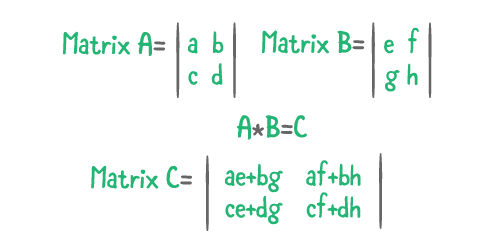There are eight recursive calls:

1. a * e
2. b * g
3. a * f
4. b * h
5. c * e
6. d * g
7. c * f
8. d * h

These combine to form the four quadrants of C.

This step alone, however, doesn’t improve the complexity. Using the Master Theorem with T(n) = 8T(n/2) + O(n²) you still get a time of O(n³).

Strassen’s insight was that you don’t actually need eight recursive calls to complete this process. You can finish the operation with seven recursive calls with a little bit of addition and subtraction.

Strassen’s seven calls are as follows:

1. a * (f – h)
2. (a + b) * h
3. (c + d) * e
4. d * (g – e)
5. (a + d) * (e + h)
6. (b – d) * (g + h)
7. (a – c) * (e + f)

Now, you can compute matrix C’s new quadrants:A great reaction right now would be !!??!?!?!!?! How does this even work?

Next, you’ll prove it!

1. First submatrix:

```p5+p4-p2+p6 = (a+d)*(e+h) + d*(g-e) - (a+b)*h + (b-d)*(g+h)
= (ae+de+ah+dh) + (dg-de) - (ah+bh) + (bg-dg+bh-dh)
= ae+bg ✅
```

Exactly what you got the first time!

Now, on to proving the others.

2. Second submatrix:

```p1+p2 = a*(f-h) + (a+b)*h
= (af-ah) + (ah+bh)
= af+bh ✅
```

3. Third submatrix:

```p3+p4 = (c+d)*e + d*(g-e)
= (ce+de) + (dg-de)
= ce+dg ✅
```

4. Fourth submatrix:

```p1+p5-p3-p7 = a*(f-h) + (a+d)*(e+h) - (c+d)*e - (a-c)*(e+f)
= (af-ah) + (ae+de+ah+dh) -(ce+de) - (ae-ce+af-cf)
= cf+dh ✅
```

Great! The math checks out!

## Implementing in Swift

Now, to the implementation! Start by adding the following extension to the bottom of your playground:

```extension Matrix {
public func strassenMatrixMultiply(by other: Matrix) -> Matrix {
// More code to come!
}
}
```

Now, just like in the naive implementation, you need to check that the first matrix’s column count is equal to the second matrix’s row count.

Replace the comment with the following:

```precondition(columnCount == other.rowCount, """
Two matrices can only be matrix multiplied if the first column's count is \
equal to the second's row count.
""")
```

Time for some prep work! Add the following right below `precondition`:

```// 1
let n = Swift.max(rowCount, columnCount, other.rowCount, other.columnCount)
// 2
let m = nextPowerOfTwo(after: n)

// 3
var firstPrep = Matrix(rows: m, columns: m)
var secondPrep = Matrix(rows: m, columns: m)

// 4
for index in indices {
firstPrep[index.row, index.column] = self[index]
}
for index in other.indices {
secondPrep[index.row, index.column] = other[index]
}
```

Reviewing what’s going on here, you:

1. Calculate the max count of the first or second matrix’s rows or columns.
2. Find the next power of two after that number.
3. Create two new matrices whose rows and columns are equal to the next power of two.
4. Copy the elements from the first and second matrices into their respective prep matrices.

This seems like extra work: Why is this necessary? Great question! Next, you’ll investigate with an example.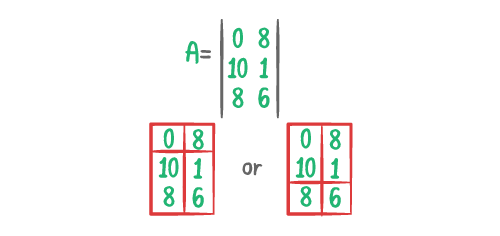Say you have a 3×2 matrix, A. How should you split this up? Should the middle row go with the top split or the bottom? Because there’s no even way to split this matrix, this edge case would need to be explicitly handled. While this seems difficult, the above prep work will remove this possibility completely.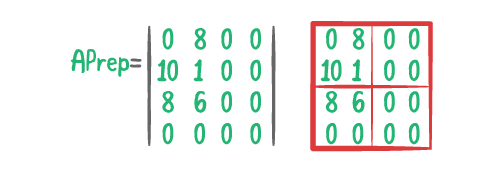By increasing the size of the matrix until it is a square matrix whose rows/columns are an even power of two, you ensure the edge case will never occur. Additionally, because the prep work only adds rows and columns with zeros, the result won’t change at all. 🎉

Now, to finish the method, add the following to `strassenMatrixMultiply`:

```// 1
let resultPrep = firstPrep.strassenRecursive(by: secondPrep)
// 2
var result = Matrix(rows: rowCount, columns: other.columnCount)
// 3
for index in result.indices {
result[index] = resultPrep[index.row, index.column]
}
// 4
return result
```

Here, you:

1. Recursively compute the result matrix.
2. Initialize a new matrix with the correct dimensions.
3. Iterate through the result matrix and copy over the identical index from the prep matrix.
4. Finally, you return the result!

Good job! Almost done. You have two unimplemented methods left, `nextPowerOfTwo` and `strassenRecursive`. You’ll tackle those now.

## nextPowerOfTwo

Add the following method below `strassenMatrixMultiply`:

```private func nextPowerOfTwo(after n: Int) -> Int {
// 1
let logBaseTwoN = log2(Double(n))
// 2
let ceilLogBaseTwoN = ceil(logBaseTwoN)
// 3
let nextPowerOfTwo = pow(2, ceilLogBaseTwoN)
return Int(nextPowerOfTwo)
}
```

This method takes a number and returns the next power of two after that number if that number is not already an even power of two.

Reviewing, you:

1. Calculate the log base 2 of the inputed number.
2. Take the ceiling of `logBaseTwoN`. This rounds the `logBaseTwoN` up to the nearest whole number.
3. Calculate 2 to the `ceilLogBaseTwoN` power and convert it to an `Int`.

### Challenge

To get a better idea of how this method works, try applying it to the following numbers. Don’t use code! Write out each step and use WolframAlpha to do the calculations.

1. 3
2. 4

[spoiler title=”Solution”]
For 3:

```log2(3) = 1.584
ceil(1.584) = 2
pow(2, 2) = 4
nextPowerOfTwo = 4
```

For 4:

```log2(4) = 2
ceil(2) = 2
pow(2, 2) = 4
nextPowerOfTwo = 4
[/spoiler]
```

## strassenRecursive

Next up, you need to implement `strassenRecursive(by other:)`. Start by adding the following below `nextPowerOfTwo`:

```private func strassenRecursive(by other: Matrix) -> Matrix {
assert(isSquare && other.isSquare, "This method requires square matrices!")
guard rowCount > 1 && other.rowCount > 1 else { return self * other }
}
```

Here, you set the base case for the recursion: If either matrix has a row length of 1, then you just return the term-by-term multiplication of the two matrices.

Then, you need to split the input matrices into 8 submatrices. Add this initialization to the method:

```// 1
let n = rowCount
let nBy2 = n / 2

//  Assume submatrices are allocated as follows
//  matrix self = |a b|,    matrix other = |e f|
//                |c d|                    |g h|

// 2
var a = Matrix(rows: nBy2, columns: nBy2)
var b = Matrix(rows: nBy2, columns: nBy2)
var c = Matrix(rows: nBy2, columns: nBy2)
var d = Matrix(rows: nBy2, columns: nBy2)
var e = Matrix(rows: nBy2, columns: nBy2)
var f = Matrix(rows: nBy2, columns: nBy2)
var g = Matrix(rows: nBy2, columns: nBy2)
var h = Matrix(rows: nBy2, columns: nBy2)

// 3
for i in 0..<nBy2 {
for j in 0..<nBy2 {
a[i, j] = self[i, j]
b[i, j] = self[i, j+nBy2]
c[i, j] = self[i+nBy2, j]
d[i, j] = self[i+nBy2, j+nBy2]
e[i, j] = other[i, j]
f[i, j] = other[i, j+nBy2]
g[i, j] = other[i+nBy2, j]
h[i, j] = other[i+nBy2, j+nBy2]
}
}
```

OK! You:

1. Initialize two variables that are the size of the current matrix and the size of the submatrices.
2. Initialize all eight submatrices.
3. Update each of the eight submatrices with the appropriate elements from the original matrices. A really cool optimization here is that you only need to loop from `0..<nBy2` instead of `0..<n`. Because the eight submatrices have the same indices, you're able to update all eight each step through the `for` loop!

Next, add the following to the bottom of the method:

```let p1 = a.strassenRecursive(by: f-h)       // a * (f - h)
let p2 = (a+b).strassenRecursive(by: h)     // (a + b) * h
let p3 = (c+d).strassenRecursive(by: e)     // (c + d) * e
let p4 = d.strassenRecursive(by: g-e)       // d * (g - e)
let p5 = (a+d).strassenRecursive(by: e+h)   // (a + d) * (e + h)
let p6 = (b-d).strassenRecursive(by: g+h)   // (b - d) * (g + h)
let p7 = (a-c).strassenRecursive(by: e+f)   // (a - c) * (e + f)
```

Here, you recursively compute the seven matrix multiplications required by Strassen's algorithm. They are the exact same seven you saw in the section above!

```let result11 = p5 + p4 - p2 + p6         // p5 + p4 - p2 + p6
let result12 = p1 + p2                   // p1 + p2
let result21 = p3 + p4                   // p3 + p4
let result22 = p1 + p5 - p3 - p7         // p1 + p5 - p3 - p7
```

Above, you compute the submatrices of the result matrix.  Now for the final step! Add the following:

```var result = Matrix(rows: n, columns: n)
for i in 0..<nBy2 {
for j in 0..<nBy2 {
result[i, j]           = result11[i, j]
result[i, j+nBy2]      = result12[i, j]
result[i+nBy2, j]      = result21[i, j]
result[i+nBy2, j+nBy2] = result22[i, j]
}
}

return result
```

Phew! Good work. In the final step, you combine the four submatrices into your result matrix. Notice that you only need to loop from
`0..<nBy2` because, in each iteration of the loop, you can fill four elements of final result matrix. Yay for efficiency!

### Example

To illustrate how the divide and conquer recursion works, look at the following the example: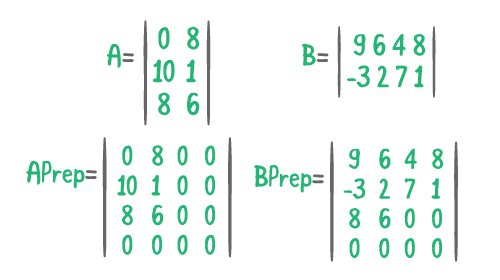Above are two matrices, a 3x2 matrix A and a 2x4 matrix B. You'll use `strassenMatrixMultiply(by:)` to calculate the matrix multiplication. Following `strassenMatrixMultiply(by:)`, first you prep the matrices, A & B into 2 4x4 matrices.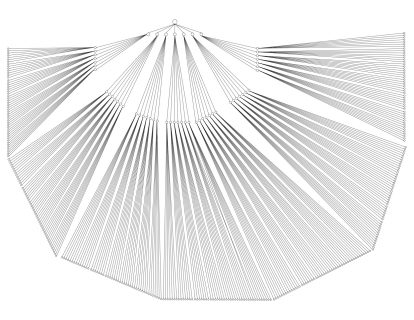Once the matrices are prepped, you begin calling the recursive portion of the algorithm, `strassenRecursive(by:)`. Above is the full recursion tree for `strassenRecursive(by:)` for this example. Each call to `strassenRecursive(by:)` generates 7 additional calls, each with a matrix half the size of the inputed matrix. This is the heart of Strassen's Algorithm, and where the divide and conquer strategy is used. You recursively split the matrices in two halves, and the solve (conquer) each bit before combining the results.

### Challenge

How many calls are there to `strassenRecursive(by:)` for this example?

[spoiler title="Solution"]

(7³) + 1 = 344. You get 7 because each call to `strassenRecursive(by:)` generates 7 additional calls. The n³ comes from the 3 layers of recursion (4 -> 2 -> 1). Finally, you need to add one to account for the first call, from `strassenMatrixMultiply(by:)`.
[/spoiler]

Next, you'll review the first branch in detail.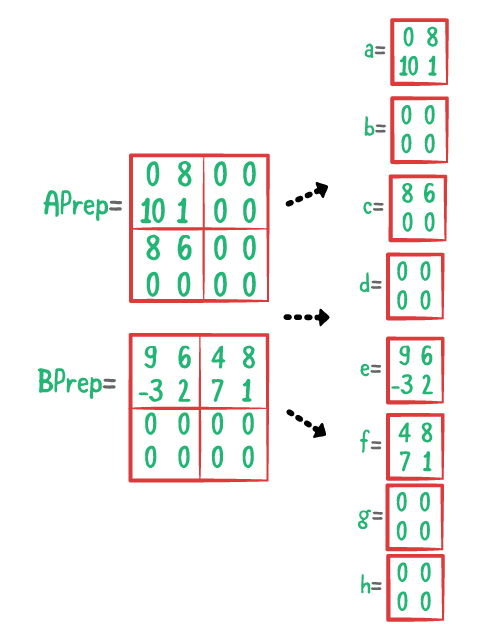Following the first call to `strassenRecursive(by:)`, you first split the matrices APrep and BPrep into 8 submatrices.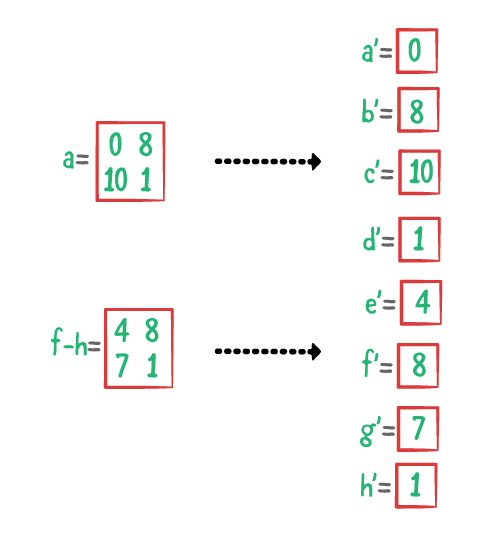You'll follow the first branch, `a.strassenRecursive(by: f-h)`. This time `self = a` and `other = f - h`. You'll then split into the 8 submatrices again.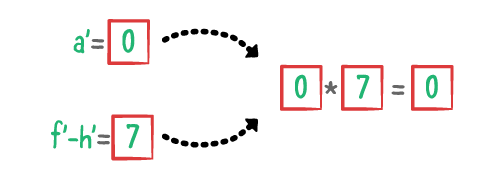As before, follow the first branch, `a.strassenRecursive(by: f-h)`. This time `self = a'` and `other = f' - h'`. Recall the line at the beginning of `strassenRecursive(by:)`:

```guard rowCount > 1 && other.rowCount > 1 else { return self * other }
```

Now, because the matrices only have one row/column, you just multiply the two elements together and return the result!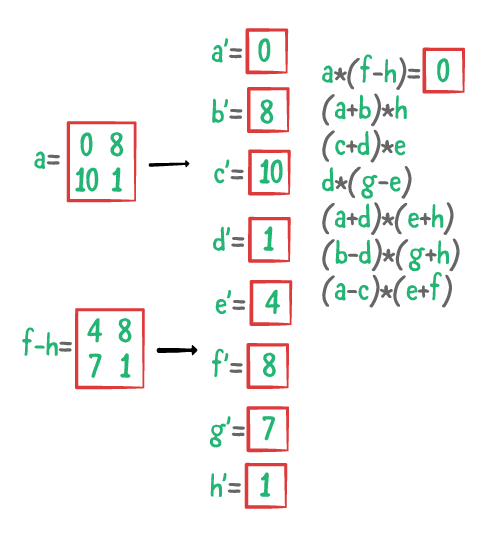The result then propagates upwards and is used in the previous recursion.

You could repeat this procedure for each and every recursion but that might take all day 😅. Good thing computers are much faster!

## Time Complexity

As before, you can analyze the time complexity using the Master TheoremT(n) = 7T(n/2) + O(n²) which leads to O(n^log(7)) complexity. This comes out to approximately O(n^2.8074) which is better than O(n³). 😁💯🙌

### Trying It Out

Now that you've done all this work, try the method out! Add the following to the bottom of your playground:

```let G = A.strassenMatrixMultiply(by: B)
printMatrix(G, name: "G")
```

If you remember from above, you actually ran this multiplication before, in matrix C. Check your answer to ensure the two agree. The output should look like:

```Matrix G:
9 7
20 10
```

Now, one more. Add the following to the bottom of your playground:

```let H = B.matrixMultiply(by: A)
printMatrix(H, name: "H")
let I = B.strassenMatrixMultiply(by: A)
printMatrix(I, name: "I")
```

```Matrix H:
6 43 -3 0
4 12 -2 0
-2 19 1 0
4 72 -2 0

Matrix I:
6 43 -3 0
4 12 -2 0
-2 19 1 0
4 72 -2 0
```

### Challenge

1. Initialize the following matrices J and K:

```Matrix J:
1 2 3 8 -1
-1 18 2 0 1

Matrix K:
-1 2 98
3 4 4
0 1 2
9 6 5
3 1 -5
```

2. Compute L, the result of applying `matrixMultiply` to J and K.
3. Compute M, the result of applying `strassenMatrixMultiply` to J and K.
4. Print L and M to the console to check that they are equal.

[spoiler="Solution"]

```// 1
var J = Matrix<Int>(rows: 2, columns: 5)
J[row: 0] = [1, 2, 3, 8, -1]
J[row: 1] = [-1, 18, 2, 0, 1]

var K = Matrix<Int>(rows: 5, columns: 3)
K[column: 0] = [-1, 3, 0, 9, 3]
K[column: 1] = [2, 4, 1, 6, 1]
K[column: 2] = [98, 4, 2, 5, -5]

// 2
let L = J.matrixMultiply(by: K)
// 3
let M = J.strassenMatrixMultiply(by: K)
// 4
printMatrix(L, name: "L")
printMatrix(M, name: "M")
```

[/spoiler]

And that's the advantage of Strassen's algorithm!

## Where to Go From Here?

You'll find the completed playground in the Download Materials button at the top or bottom of the tutorial. It has all the code you've already implemented above. You can also find the original implementation and further discussion in the Strassen's Matrix Multiplication section of the Swift Algorithm Club repository.

For more practice with divide and conquer algorithms, check out Karatsuba Multiplication or Merge Sort, both of which are implemented in the Swift Algorithm Club repository.

If you're interested in faster matrix multiplication algorithms, look at Coppersmith–Winograd algorithm. It's the faster known matrix multiplication algorithm and has about a O(n^2.372) complexity.

This was just one of the many algorithms in the Swift Algorithm Club repository. If you're interested in more, check out the repo.

It's in your best interest to know about algorithms and data structures — they're solutions to many real-world problems and are frequently asked as interview questions. Plus, they're fun!

Stay tuned for more tutorials from the Swift Algorithm Club in the future. In the meantime, if you have any questions on implementing Strassen's algorithm in Swift, please join the forum discussion below!

Note: The Swift Algorithm Club is always looking for more contributors. If you've got an interesting data structure, algorithm, or even an interview question to share, don't hesitate to contribute! To learn more about the contribution process, check out our Join the Swift Algorithm Club article.